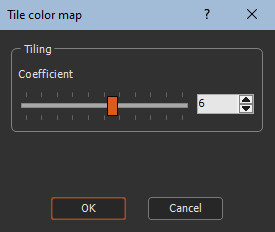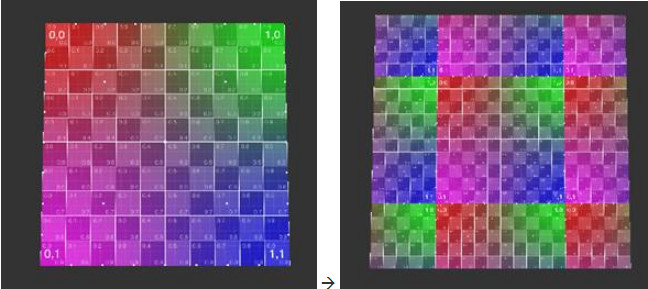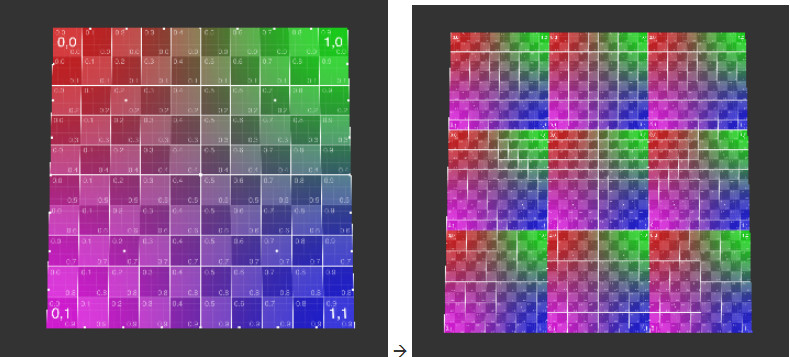### Adding a Tile color map node

This node takes an input color map and generates a color map that corresponds to N x N times the starting color map with N being the repetition coefficient between 1 and 10.

To add a node, right click in the Graph Editor and select Create Node  > Color  > Tile color map.### Editing a tile color map node

Use the slider to set the tiling coefficient.For example, changing the coefficient from 1 to 2 gives the following results.Changing the coefficient from 1 to 3 gives the following results.A high coefficient can lead to a high resolution color map. For example, if the input color map has a size of 1000 x 1000 and the repetition coefficient is equal to 8, then the output color map will have a size of 8000 x 8000.